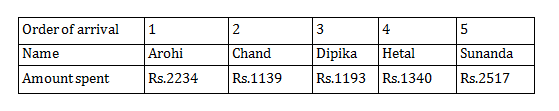Direction for questions 1- 3: Answer the questions based on the following information.
Five women decided to go for shopping to South Extension, NewDelhi. They arrived at the designated meeting place in the following order: 1. Arohi, 2. Chand, 3. Dipika, 4. Hetal, and 5. Sunanda. Each of them spent atleast Rs, 1000. The woman who spent Rs. 2234 arrived before the woman who spent Rs. 1193. One of them spent Rs. 1340 and she was not Dipika. One woman spent Rs. 1378 more than Chand. One of them spent Rs. 2517 and she was not Arohi. Hetal spent more than Dipika. Sunanda spent the largest amount and Chand the smallest.

Question 1 : Which of the following amount is spent by one of the women?
A. Rs. 1139
B. Rs. 1378
C. Rs. 2518
D. Rs. 2571

Question 2 : What was the amount spent by Hetal?
A.Rs. 2234
B. Rs. 1340
C. Rs. 1193
D. Rs.2517

Question 3 : The lady who spent Rs. 1193 is:
A.Hetal
B. Chand
C. Dipika
D. Arohi

Answers: For questions 1 to 3: Four of the amounts spent by the five women are Rs.2234, Rs.1193, Rs.1340 ad Rs.2517

Two cases arise: (i) The lowest amount spent is Rs. 1193 (by Chand): Then, the fifth amount will be Rs.(1193 + 1378) = Rs. 2571, which will then be the highest amount and is spent by Sunanda. As Arohi arrived before Chand, so she must have spent Rs.2234. This implies Hetal spent Rs.2517 and Dipika spent Rs.1340, which is a contradiction. Hence, this case is not possible. (ii) The highest amount spent is Rs.2517 (by Sunanda): Then the fifth amount will be Rs.(2517 – 1378) = Rs.1139. Since it is the lowest amount, it will be spent by Chand. Further analysis leads to the following table:Answer 1 : (A)

Answer 2 : (B)

Answer 3 : (C)